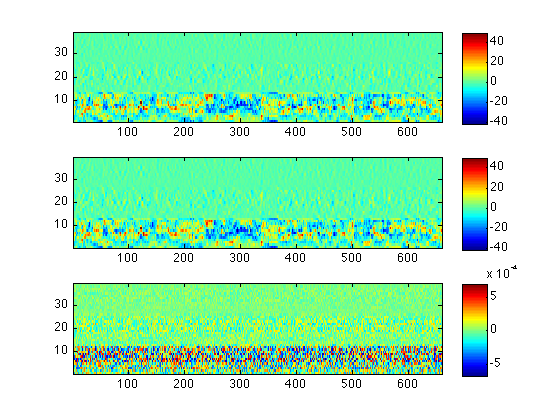LabROSA : Projects:# calc_mfcc - Emulation of HTK's MFCC calculation

calc_mfcc is a (compiled) Matlab script that calculates MFCC features, attempting to duplicate a particular configuration of MFCC's HCopy.

The code is based on my rastamat package that attempts to mimic a lot of well-known MFCC variants, but this version has a few extra tweaks to make it closer to HTK output.

The specific HTK configuration we're aiming to copy is this:

``` TARGETRATE = 100000.0
SOURCEFORMAT = WAV
TARGETKIND = MFCC_0_D_A_Z
ENORMALISE = T
USEPOWER = T
ZMEANSOURCE = T
SAVECOMPRESSED = T
SAVEWITHCRC = T
USEHAMMING = T
WINDOWSIZE = 250000.0
CEPLIFTER = 22
NUMCHANS = 26
NUMCEPS = 12
BYTEORDER = VAX
PREEMCOEF = 0.97```

## Example Usage

The Matlab script can be run from the Matlab prompt, or using the included Unix shell wrapper, run_calc_mfcc.sh:

```% Run it over our demo file
calc_mfcc test.wav test.out
% Plot the result
subplot(311)
imagesc(d(:,3:end)'); axis xy
colorbar
% Compare to HTK output
subplot(312)
imagesc(h(:,3:end)'); axis xy
colorbar
% and difference
subplot(313)
imagesc(h(:,3:end)'-d(:,3:end)'); axis xy
colorbar
% Quantify
mean(mean(abs(h(:,3:end)-d(:,3:end))))/mean(mean(abs(h(:,3:end))))
```
```ans =

2.9091e-05

```## Installation

This package has been compiled for several targets using the Matlab compiler. You will also need to download and install the Matlab Compiler Runtime (MCR) Installer. Please see the table below:

ArchitectureCompiled packageMCR Installer
64 bit Linux calc_mfcc_GLNXA64.zip Linux 64 bit MCR Installer
64 bit MacOS calc_mfcc_MACI64.zip MACI64 MCR Installer

The original Matlab code used to build this compiled target is available at http://labrosa.ee.columbia.edu/projects/calc_mfcc/

All sources and data files are in the package calc_mfcc-v0.2.zip.

Feel free to contact me with any problems.

## Changelog

```% 2013-06-24 v0.2 Fix in deltas.m for zero-length segments.
%
% 2013-02-26 v0.1 Initial release
%
```

## Acknowledgment

This work was supported by DARPA under the RATS program via a subcontract from the SRI-led team SCENIC. My work was on behalf of ICSI.

\$Header: /Users/drspeech/data/RATS/code/calc_mfcc/RCS/demo_calc_mfcc.m,v 1.1 2013/02/26 13:35:11 dpwe Exp dpwe \$